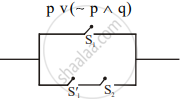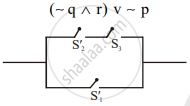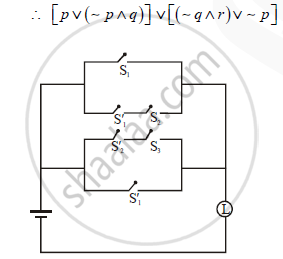Construct the Switching Circuit for the Following Statement : P V (~ P ∧ Q) V (- Q ∧ R) V ~ P - Mathematics and Statistics

Sum

Construct the switching circuit for the following statement : [p v (~ p ∧ q)] v [(- q ∧ r) v ~ p]

Solution

Let, p : Switch S1 is closed.
∼ p : Switch S1 is open.
q  : Switch S2 is closed.
∼ q : Switch S2 is open
r : Switch S3 is closed.
∼ r : Switch S3 is open.
Now,Concept: Application of Logic to Switching Circuits
Is there an error in this question or solution?